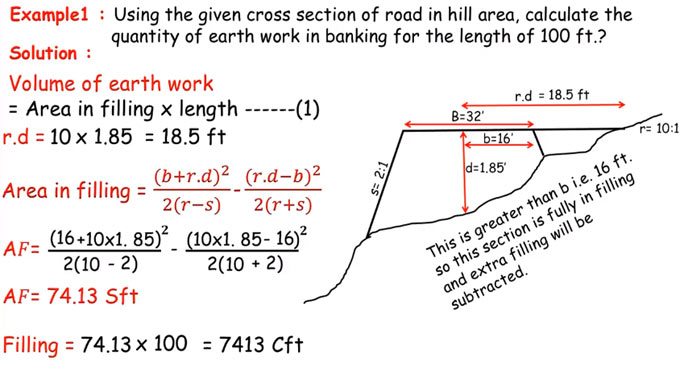# Steps to work out road filling in hilly areaS.L. Khan, the eminent civil engineer, presents another informative construction video tutorial where he briefly explains the process for working out the road filling in hilly area.

In this video, solution is given to the following examples :-

Examaple 1

With specified cross section of road in hill area, work out the quantity of earth work in banking toward the length of 100 ft.

Suppose the permission rate of the road is taken as breadth of the road and it is 32 feet. The half of it is 16 feet. The mean depth of the filling is taken as 1.85 feet. The side slope is taken as 2:1. The cross slope is taken as 10:1.

Based on the above data, the quantity of earth work in banking will be determined.

To obtain the total filling of this section, apply the following formula :-

Filling = Cross Section Area x Length

To learn rest calculation process, go through the following video tutorial.

Video Source: SL Khan